• +91 9971497814
• info@interviewmaterial.com

# RD Chapter 30- Derivatives Ex-30.3 Interview Questions Answers

### Related Subjects

Question 1 : Differentiate the following with respect to x:

x4 – 2sin x + 3 cos x

Given:

f (x) = x4 – 2sinx + 3 cos x

Differentiate on both the sides with respect to x, we get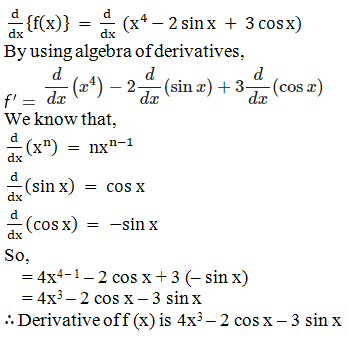Question 2 :

3x + x3 +33

Given:

f (x) = 3x + x3 + 33

Differentiate on both the sides with respect to x, we get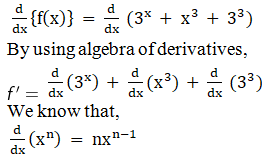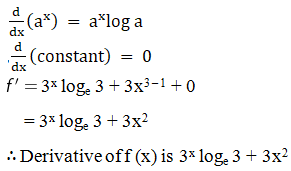Question 3 :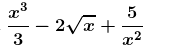Given: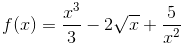Differentiate on both the sides with respect to x, we get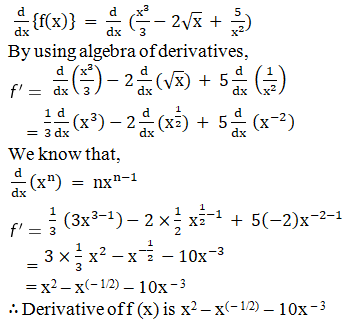Question 4 :

ex log a + ealog x + ea log a

Given:

f (x) = ex log a +ea log x + ea log a

We know that,

elog f(x) = f(x)

So,

f(x) = ax + xa + aa

Differentiate on both the sides with respect to x, we get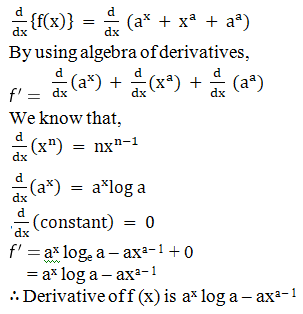Question 5 :

(2x2 + 1) (3x + 2)

Given:

f (x) = (2x2 + 1)(3x + 2)

= 6x3 + 4x2 + 3x + 2

Differentiate on both the sides with respect to x, we get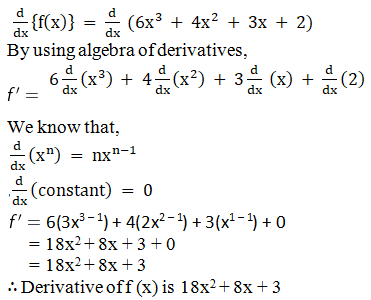Todays Deals### RD Chapter 30- Derivatives Ex-30.3 Contributorskrishan

Name:
Email:

# Latest News# 9000 interview questions in different categories